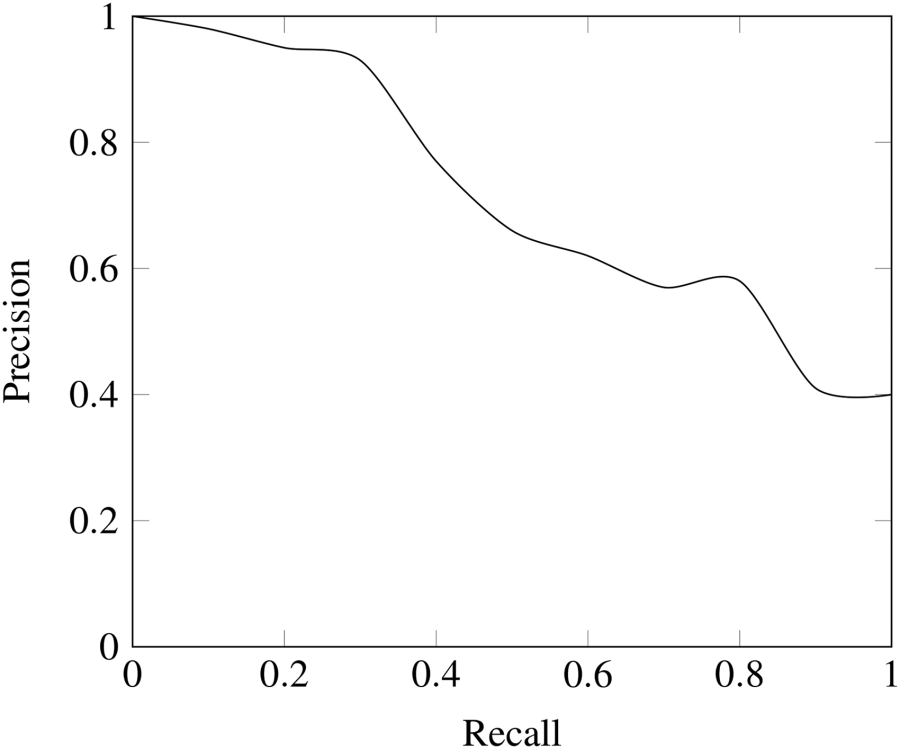# Precision and Recall

In statistics, recall refers to the ability to find all the relevant cases within a dataset. Mathematically it is defined as the number of true positives over the sum of the true positives and false negatives.

(for a refresher on true/false positives/negatives, see type I and type II errors)

One will find that simply labelling all data points as positives will maximise recall, reaching a value of 1.0. This is of course, at the expense of precision.

Precision refers to the ability to find only the relevant cases within a dataset. Mathematically it is defined as the number of true positives over the sum of the true positives and false positives.

This is better understood via an example. Suppose we want to classify whether each passenger of a plane has dietary requirements ahead of time to optimize their booking flow.

This is a binary classification for each passenger, which is greatly unbalanced towards negatives (i.e., no, this passenger does not have dietary requirements), which makes it a good problem for a precision & recall metric.

If we simply classify all passengers as not having dietary requirements, then we will have 0 precision and 0 accuracy, assuming there are at least some passengers with requirements.

If we simply classify all passengers as having dietary requirements, then we will have very high recall, but low precision.

If we successfully classify a single passenger as having dietary requirements, but there are several more that do, we will have high precision, but low recall.

This is why it is important to find a good balance between precision and recall, which we can inspect via a precision and recall plot.